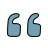### Development of Reasons for Classroom Disengagement Scale: Reliability and Validity Study

###### Author:

Number of pages: 900-918
Year-Number: 2021-Volume 13, Issue 3

### Abstract

In this study, it was aimed to develop a scale that will be used to determine the reasons for not participating in the classes (SRNPC) for secondary school students. In the study, exploratory sequential design, one of the mixed method designs, was used. In the research, firstly, student and teacher opinions were consulted and a draft scale form was prepared regarding the reasons for not participating in the classes. Then, the scale was applied to the study group, and the validity and reliability analyses of the obtained data were carried out. Within the scope of validity analysis of the data; firstly exploratory factor analysis (EFA) was applied and a scale consisting of 21 items and four factors was obtained. The total variance of the cumulative variance for eigenvalues ​​of these four factors was found to be 52.98%. Within the scope of reliability analysis, Cronbach alpha analysis was applied, the total alpha value was found as 0.88; and the alpha values ​​of the factors were found as 0.82, 0.75, 0.75 and 0.74, respectively. The results of the t test based on the lower-upper group mean difference, item-total analysis and correlation analysis between factors were also found to be significant. As a result of the correlation analysis, it was determined that there was a positive significant relationship between the factors. Finally, confirmatory factor analysis (CFA) was applied to evaluate the compatibility of the factors with the real data and to test whether the factors were sufficient to explain the model. As a result of the DFA analysis, it was found that the factor loads varied between .41 and .99 and all of them were significant. It was determined that the model fit indices were at an acceptable level. In addition, it was found that the covariance values between factors were significant and there was a positive relationship between the factors. According to the findings obtained, it was determined that the SRNPC was a valid and reliable tool.

### Keywords

• Article Statistics
•Quote